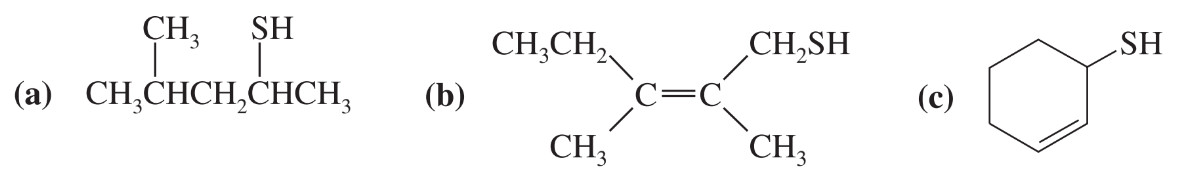×
Get Full Access to Organic Chemistry - 8 Edition - Chapter 10 - Problem 28p
Get Full Access to Organic Chemistry - 8 Edition - Chapter 10 - Problem 28p

×

# Give IUPAC names for the following compounds.ISBN: 9780321768414 33

## Solution for problem 28P Chapter 10

Organic Chemistry | 8th Edition

• Textbook Solutions
• 2901 Step-by-step solutions solved by professors and subject experts
• Get 24/7 help from StudySoup virtual teaching assistantsOrganic Chemistry | 8th Edition

4 5 1 414 Reviews
20
3
Problem 28P

Give IUPAC names for the following compounds.Step-by-Step Solution:
Step 1 of 3

IUPAC names:-

1. 4-methyl-2-sulfhydrylpentane
2. 2,3-dimethyl-1-sulfhydrylpent-2-ene
3. thiophenol
Step 2 of 3

Step 3 of 3

## Discover and learn what students are asking

Calculus: Early Transcendental Functions : Riemann Sums and Definite Integrals
?In Exercises 3–8, evaluate the definite integral by the limit definition. $$\int_{1}^{2}\left(x^{2}+1\right) d x$$

Statistics: Informed Decisions Using Data : Inference about the Difference between Two Medians: Independent Samples
?In Problems 1–8, use the Mann–Whitney test to test the given hypotheses at the = 0.05 level of significance. The independent samples were obtained ra

Unlock Textbook Solution